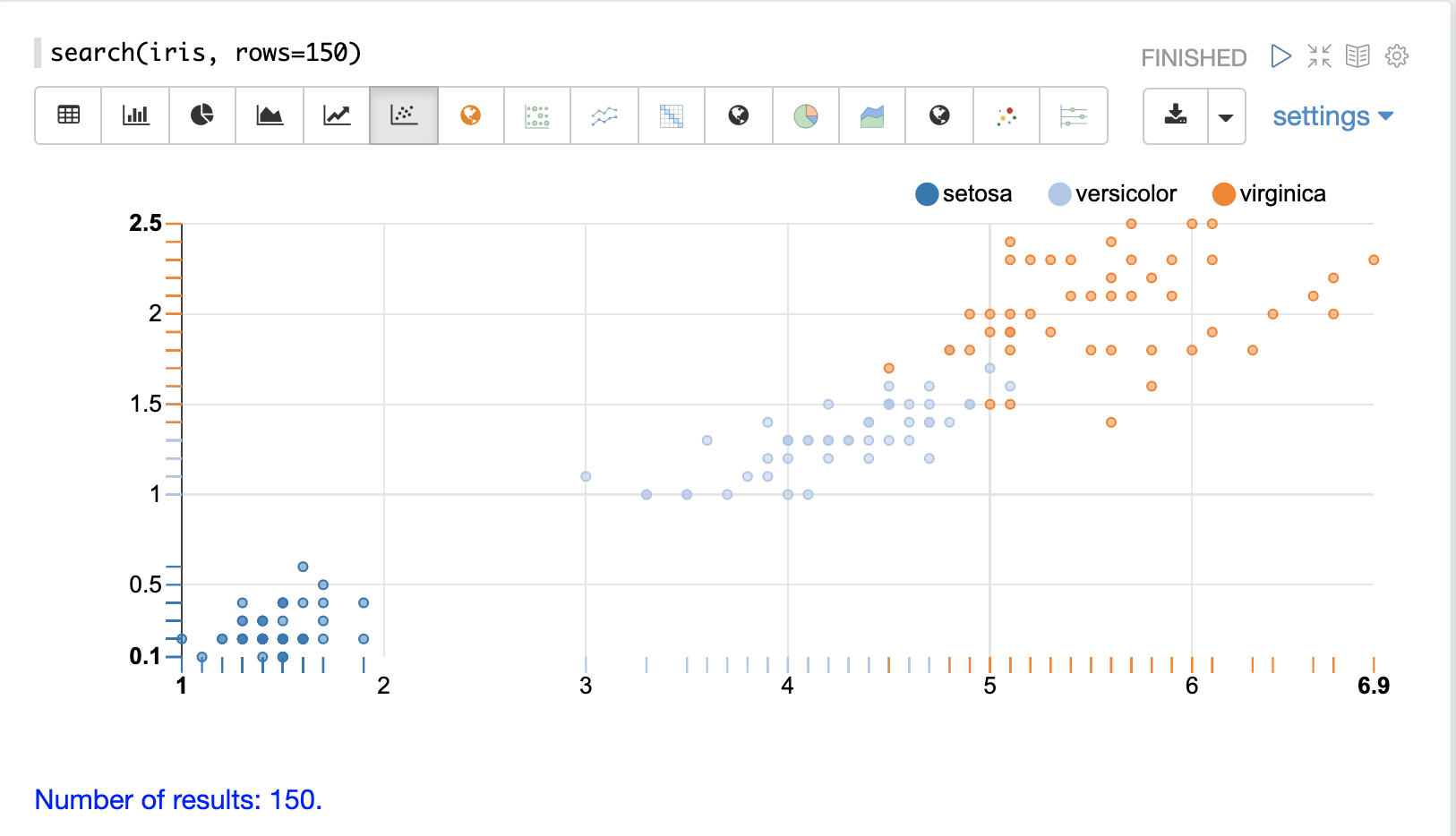# Streaming Expressions and Math Expressions

## A Visual GuideVisualizations: Gallery of streaming expression and math expression visualizations.

Getting Started: Getting started with streaming expressions, math expressions, and visualization.

Searching, Sampling and Aggregation: Searching, sampling, aggregation and visualization of result sets.

Transforming Data: Transforming and filtering result sets.

Scalar Math: Math functions and visualization applied to numbers.

Vector Math: Vector math, manipulation and visualization.

Variables and Vectorization: Vectorizing result sets and assigning and visualizing variables.

Matrix Math: Matrix math, manipulation and visualization.

Text Analysis and Term Vectors: Text analysis and TF-IDF term vectors.

Probability: Continuous and discrete probability distribution functions.

Statistics: Descriptive statistics, histograms, percentiles, correlation, inference tests and other stats functions.

Linear Regression: Simple and multivariate linear regression.

Curve Fitting: Polynomial, harmonic and Gaussian curve fitting.

Time Series: Time series aggregation, visualization, smoothing, differencing, anomaly detection and forecasting.

Interpolation and Numerical Calculus: Interpolation, derivatives and integrals.

Signal Processing: Convolution, cross-correlation, autocorrelation and fast Fourier transforms.

Simulations: Monte Carlo simulations and random walks

Machine Learning: Distance, KNN, DBSCAN, K-means, fuzzy K-means and other ML functions.

Computational Geometry: Convex Hulls and Enclosing Disks.

Appendix A: Solr log analytics and visualization.